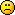Discussion about math, puzzles, games and fun.   Useful symbols: ÷ × ½ √ ∞ ≠ ≤ ≥ ≈ ⇒ ± ∈ Δ θ ∴ ∑ ∫  π  -¹ ² ³ °

You are not logged in.

## #1 2018-12-04 21:10:55

Spicca
Member
Registered: 2018-12-04
Posts: 1

### Probability function

Pick 4 random points on a sphere. what Probability function has the volume of tetrahedron with these points?

I've seen the question of average volume from somewhere and I am interested in the probabilities of the volume. (unfortunately I couldn't understand the answer...)

I'm sorry if my english is bad. If you don't understand anything please let me know. Thank you!

Offline

## #2 2018-12-10 16:45:29

mr.wong
Member
Registered: 2015-12-01
Posts: 252

### Re: Probability function

Hi  Spicca ,

I  really  cannot  solve  this  problem . Or  we  may  consider  a  similar  but  simpler  one  of  2- dimensional  ,  perhaps  we  can  get  some  hint .

From  a  circle  with  radius  1  unit , 3  points  are  picked  randomly  on  its  circumference . Find  the  expected  area  of  the  triangle  so  formed .

Offline

## #3 2018-12-22 19:01:27

mr.wong
Member
Registered: 2015-12-01
Posts: 252

### Re: Probability function

For  the  problem  of  2-dimensional  , let  O  be  the  centre  of  the  circle  . Take  a  point  A  on  the  circumference  ,  and  let  AD  be  the  diameter  with  length  2  unit . If  we  take  2  more  points  B  and  C  randomly  on  the  circumference  to  form  a  triangle ,  the  chance  that  B  and  C  lie  on  the  same  side  of  AD  is  1/2 ,  while  the  chance  that  B  and  C  lie  on  the  opposite  sides  of  AD  is  also  1/2 . We  shall  consider  the  1st  case  firstly . Let  x  be  the  length  of  AB , and  let  y  be  the  length  of  AC . ( For  simplicity  let  x ≤ y ) Then  both  x  and  y  varies  from  0  to  2  units .
We  know  that  the  area  of  Δ ABC  =  Δ ABO  +  Δ OBC  -  Δ ACO .  But  how  to  find  the  areas  of  the  last  3   Δ s  ?

Offline

## #4 2018-12-23 03:07:09

bob bundyRegistered: 2010-06-20
Posts: 8,462

### Re: Probability function

hi Spicca

Welcome to the forum.

I've been hoping that someone would post an answer to this and I could learn too.  No such luckSo I'm having a go myself.  Please note: I've never done this before, so what follows may be rubbish.  Please comment …. ask for clarification  … tell me why it's wrong etc.  Maybe between us, we can arrive at the correct answer.  And oh yes … your English is good.

So let's work with 3D coordinates with the x-y plane horizontal and z going straight up.  Further, let's make the sphere have unit radius (cannot see any harm in that) and centred on the origin, O.

If P is one point of the tetrahedron, then we can specify its position using spherical coordinates theta and phi as shown here: https://en.wikipedia.org/wiki/Spherical … ate_system  And let P' be the point in the x-y plane below P.

Now, what would be helpful is to have a formula for the volume of a tetrahedron in terms of theta and phi but I cannot find one.  Plenty of internet pages giving the formula in vector terms such as https://math.stackexchange.com/question … ot-product

and as a determinant https://stackoverflow.com/questions/986 … n-4-points

I could also expand either into a large algebraic formula but it would take ages to enter all the LaTex so you'll have to ask nicelyif you want this.

phi can take any random value from 0 to 2pi and theta any from 0 to pi.

So you can construct your function with 8 variables and there it is.  Hhhmmmm.Bob

Children are not defined by school ...........The Fonz
You cannot teach a man anything;  you can only help him find it within himself..........Galileo Galilei
Sometimes I deliberately make mistakes, just to test you!  …………….Bob BundyOffline